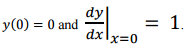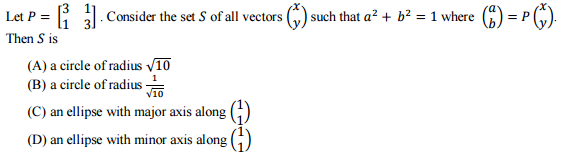# GATE Answer Key 7th Feb 2016: Electrical Branch

Q.30 Let y(x) be the solution of the differential equationwith initial conditionsThen the value of y(1) is _________.

Q.31Q.32Q.33 Let the probability density function of a random variable, ܺ, be given as:
where u(x) is the unit step function.
Then the value of 'a' and ܲProb {x<= 0}, respectively, are
(A) 2, 1/2
(B) 4, 1/2
(C) 2, 1/4
(D) 4, 1/4
Please report if you find any errors in the questions.
Answers/Solution of remaining questions are under evaluation and the same will be published here soon.
Keep watching the space←Previous
« Prev Post
Next→
Next Post »

1.2.Q 31 ?
how did u find out?

3.4.5.